# Optimization of Biodiesel Production

By Rutvik Solanki
Published in Projects

The fuel crisis is a world-renowned problem and the search for alternative sources of energy is of high necessity currently. Biodiesel is an excellent option as it theoretically gives overall zero carbon emission and just needs a few modifications in the current engines to work as fuel. This article analyzes the process for producing Biodiesel from oils. It uses a mathematical model for simulating the reaction and optimizing the Bio-diesel yield. Further, an approach to generalize the process of parameter tuning and optimizing the yield for any oil is discussed in the paper. The main aim is to develop a framework that can be integrated as open-source software for biodiesel optimization of any oil with just one calibration dataset.

The reaction of oil (TG) and solution of KOH in methanol (M) proceeds according to a simple stoichiometric equation:The reaction of rapeseed oil (RO) and solution of KOH in methanol (M) proceeds according to a simple stoichiometric equation: RO + 3 M = G + 3 E (1) With G = glycerol and E = methylic esters of RO biodiesel. However, the physicochemical mechanism of the reaction is complicated because:

1. Rapeseed oil is a large-scale mixture of more than 100 substances. Its main part consists of triacylglycerols (TG) of four fatty acids (FA), namely oleic, linoleic, linolenic, and palmitic acid. The rest (only a few wt-%) is composed of diacylglycerols (DG) and monoacylglycerols (MG) of these FA, free FA, water (W), and traces of other substances.
2. Except for the main process, i.e. the methanolysis, all present substances can take part in various different reactions with the methanolic solution of KOH. The dominant side reactions are the saponification of TG, DG, MG, and E as well as the neutralization of free FA by KOH (salification).
3. Methanolysis of rapeseed oil is a set of simultaneous reactions and is heterogeneous during the whole course. The reaction mixture consists in each case of two (or more) liquid phases]. As the reaction mixture is very heterogeneous, the kinetics (equilibrium) of3) Methanolysis of rapeseed oil is a set of simultaneous reactions and is heterogeneous during the whole course. The reaction mixture consists in each case of two (or more) liquid phases]. As the reaction mixture is very heterogeneous, the kinetics (equilibrium) of methanolysis can be influenced by different combinations of chemical and physical processes and states. The complete mechanism of the reaction is then given by the rate, range, and combinations of particular reaction steps. It was studied on the basis of the experimentally determined compositions of the actual and final reaction mixtures at different conditions (temperature, initial composition), considering the fact, that the reaction can be influenced by either chemical (i.e. the chemical reactions) or physical (e.g. diffusion, adsorption, reaction on phase interface, hydrodynamic conditions, density, viscosity, etc.) parameters, in the most complicated case by their combination.

In this work, we consider chemical reactions as the determining reaction parameters. Nevertheless, it was necessary to presume several simplifications. However, all of them have a real basis, as will be shown in the following.

### Proposed Simplification

For the theoretical processing of the mechanism, we assume that:

1. Of the above-mentioned four FA, only TG are present in the initial reaction mixture, thus RO = TG.
2. Only two reactions from all theoretically possible are proceeding: the methanolysis of glycerides (TG, DG, MG) with the formation of E and G and saponification of TG, DG, MG, or E by KOH with the formation of potassium salts of the mentioned FA (soaps, KA) and G or M.
3. All the present isomers of TG react in the given type of reaction (alcoholysis, saponification) with the same rate and mechanism. The same is valid for isomers of DG, MG, and E, but with different rates in every group of isomers. This simplification is possible, as methanolysis and saponification take place at the carboxyl group of the FA, whereas the velocities of these reactions depend only a little on the length of the hydrocarbon chain, number, or location of the double bonds in the various FA of the oil.
4. Methanolysis is catalyzed by OH– or CH3O– ions. Concentrations of OH– and CH3O– ions are much smaller than those of TG and M and they would stay constant if methanolysis was the only reaction taking place. The particular reaction steps may be reversible or irreversible. In saponification, OH– ions are the reactants and their concentration decreases with increasing reaction times. Formed KA practically cannot be esterified by free M, DG, or MG. Therefore saponification can be considered irreversible.

### Presumed model of the reaction of rapeseed oil and the solution of KOH in methanol

We presume a reaction scheme with two sequences of consecutive competitive reactions. The first sequence describes the main part of the process – namely methanolysis of TG to methyl esters, the second describes the side reaction – saponification of glycerides and methyl esters by the catalyst to potassium soaps. The reaction scheme is expressed by the stoichiometric equations (2-12). In the equations following abbreviations are used:

OH = OH–, X = CH3O–, X = CH3O– and A–, TG–, DG–, MG–, G– are anions of salt, TG, DG, MG and G

#### Formation of Methoxide#### Methanolysis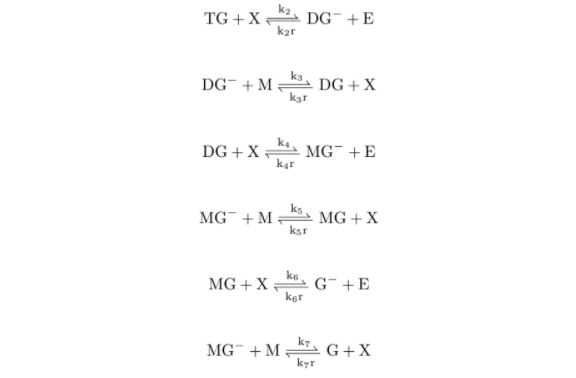#### Saponification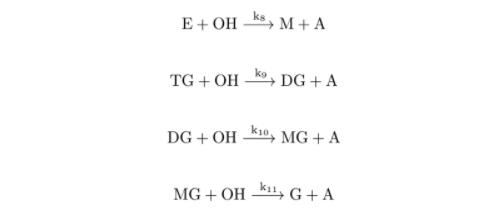whereas <−−> depicts an equilibrium (reversible) reaction, −−> indicates an irreversible (only one way) step and ki or kir are the reaction rate constants of the direct or reverse steps of the given reaction. In the reversible reaction, the ratio ki/kir defines the equilibrium constant of the given reaction ki

The irreversible step is the reaction with ki, i.e. ki, kir (at a given pressure and temperature). Its final state is characterized by the practically full exhaustion of the reactant with lower initial concentration. The scheme presumes that methanolysis of TG to G is catalyzed by methoxide X which is primarily formed by the methoxide reaction and regenerated by the reactions of Methanolysis with anions of diglycerides, monoglycerides, and glycerides (DG–, MG– and G– ). According to the methoxide equation, an important influence of water in the reaction mixture must be expected. The methanolysis proceeds in a liquid medium (i.e. at a practically constant volume) and at constant temperature and pressure. Therefore its common kinetics could be described, with respect to the rules of the formal reaction kinetics for the isothermal-isochoric reactions, by means of the molar concentrations I of all 13 assumed reactants M, OH, X, W, TG, DG–, E, DG, MG–, MG, G–, G, and A, thus by a system of 13 differential kinetic equations of the type concentration-time d[I]/DT = … . For the solution of kinetic problems, it is advantageous to express the amounts of the reactants in dimensionless relative concentrations. In our system of rate equations, the initial concentration of TG, i.e. TG = TG = a, was used as the norm for all reactants except M, X, and E.

For these components, the initial concentration of M, i.e. [M]o = b was chosen as the norm. Thus, the following relative concentrations with the values in the interval (0; 1) were defined as follows:

TG = [TG]/a; DG = [DG]/a; MG = [MG]/a; G = [G]/a; A = [A]/a; OH = [OH]/a; W = [W]/a; M = [M]/b; E = [E]/B

However, it is known that in such complicated reaction schemes rarely all reaction steps influence the real reaction rate to the same extent. Generally, some of them are dominant with respect to their great or small velocity in comparison with the other steps. We assume the same in the proposed scheme for the following reasons:

1. The alkaline-catalyzed methanolysis of the rapeseed oil takes from several tens of minutes to several hours (according to ratios n and p) at room temperature till it reaches an equilibrium. On the other hand, the formation of methanolate X, i.e. the exchange of proton between M and OH by methoxide reaction is a matter of seconds.
2. Similarly, we suppose that also all other exchanges of protons between anions DG–, MG–, G–, X and the neutral molecules M, DG, MG, G, i.e. methanolysis reactions, are in both steps much faster than the reversible transformations of TG, DG, MG by X to E and DG, MG, G, and much faster than all the irreversible saponifications. In other words, we presume that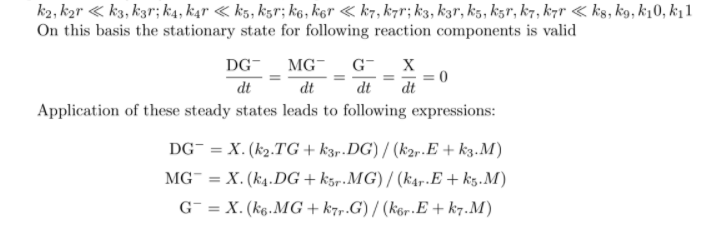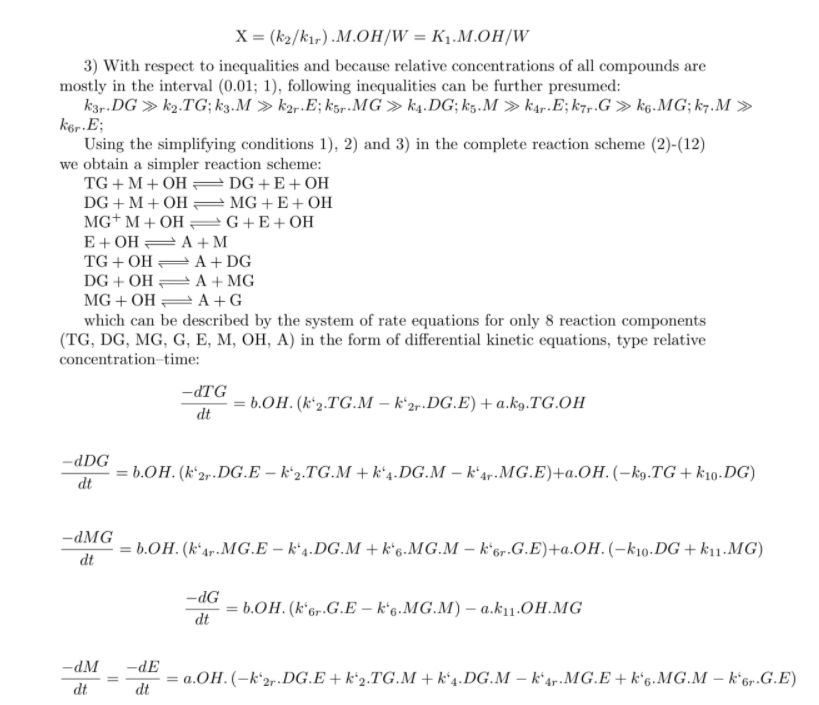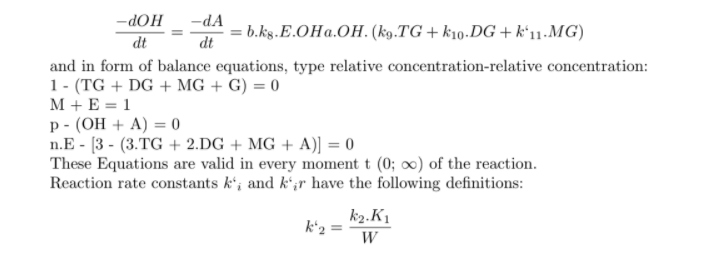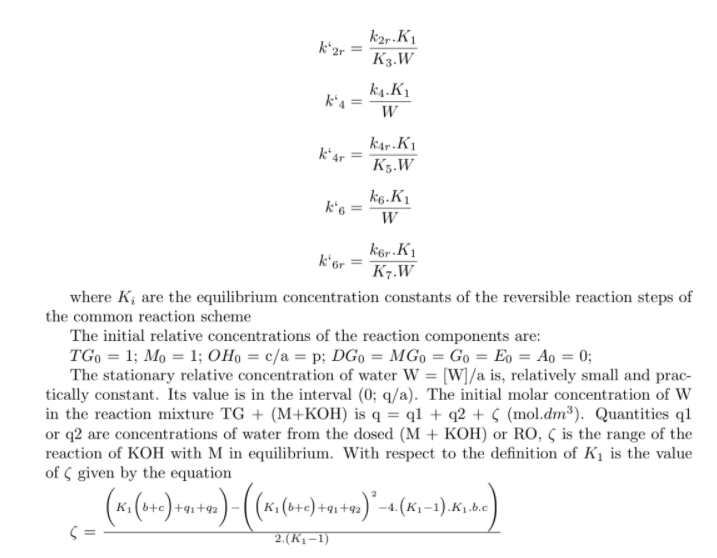The mathematical model has been coded in MatLab and has been validated against the experimental data. The code can be found here.

## Tags

Rural DevelopmentComputational Engineering
Previous Article
Thermal Analysis of a Hemi-Spherical Furnace

## Related Posts

Biodiesel - A Viable Renewable Energy Resource
4 min